### EOMONTH Function – Last Day of Month (Excel, VBA, G Sheets)

This tutorial demonstrates how to use the Excel EOMONTH Function in Excel to find the last day of the month, n months away.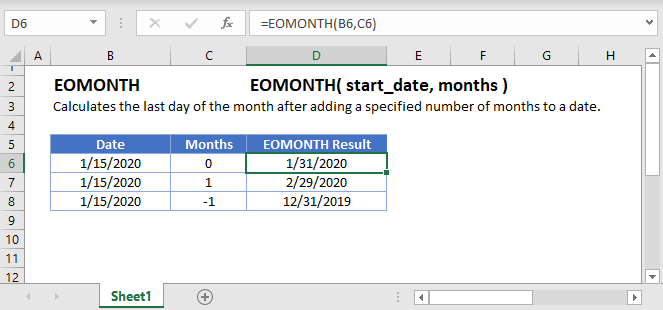## EOMONTH Function Overview

The EOMONTH Function Returns the last day of the month, n number of months away from the start date.

To use the EOMONTH Excel Worksheet Function, Select cell and Type: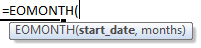(Notice how the formula input appear)

## EOMONTH Function syntax and Inputs:

start_date – The start date in Excel serial number format or entered as a date with quotations (“s) surround the date. Example: You can not enter 11/12/2015 directly into the cell. Instead you need to enter “11/12/2015” or you would need to use the corresponding serial number: 42320. Alternatively, you can reference a cell with the date 11/12/2015 entered. Excel automatically converts dates stored in cells into serial format (unless the date is entered as text).

months – Number of months away from start date. Number can be positive (future) or negative (past).

AutoMacro - VBA Code Generator

## Last Day of the Month

### Current Month

The EOMONTH Function takes a date and returns the last day of a month: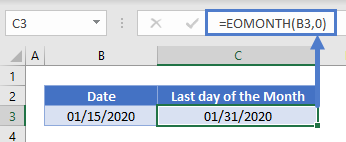### Previous Month

You can also add (or subtract) n months to the start date to get the last day of another month. This example will return the last day of the previous month: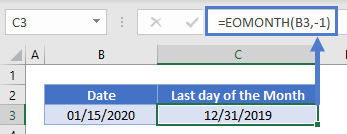## Number of Days in Month

By using the DAY Function, you can calculate the number of days in the month: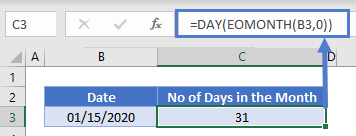The DAY Function returns the day number of a date. By using EOMONTH, we can get the last day of the month and the DAY function then returns the corresponding day number.

## First Day of Month

By adding 1 to the result of the EOMONTH Function, you can get the first day of a month: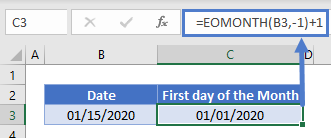Note: in Excel, dates are stored as serial numbers where each number represents a day, so adding 1 to a date, will add 1 day.

## EOMONTH in Google Sheets

The EOMONTH Function works exactly the same in Google Sheets as in Excel: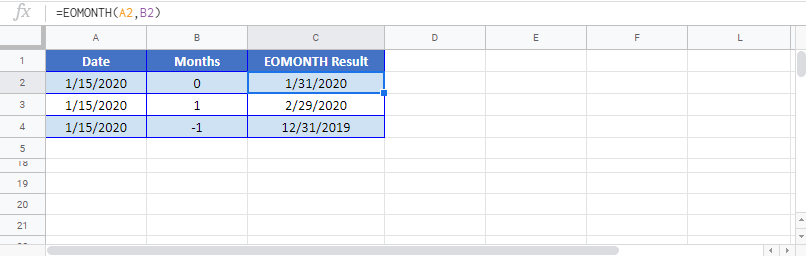## EOMONTH Examples in VBA

You can also use the EOMONTH function in VBA. Type:` application.worksheetfunction.eomonth(start_date,months)`

Running the following VBA statements

Will produce the following results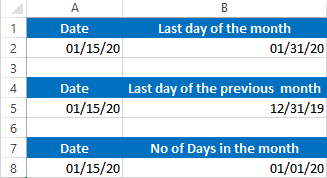For the function arguments (start_date, etc.), you can either enter them directly into the function, or define variables to use instead.

Return to the List of all Functions in Excel

## Excel Practice Worksheet

Practice Excel functions and formulas with our 100% free practice worksheets!

• Automatically Graded Exercises
• Learn Excel, Inside Excel!